Atmos. Chem. Phys., 20, 5513–5526, 2020
https://doi.org/10.5194/acp-20-5513-2020
Atmos. Chem. Phys., 20, 5513–5526, 2020
https://doi.org/10.5194/acp-20-5513-2020

Research article 12 May 2020

Research article | 12 May 2020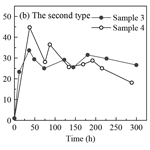# Partitioning of hydrogen peroxide in gas-liquid and gas-aerosol phases

Partitioning of hydrogen peroxide in gas-liquid and gas-aerosol phases
Xiaoning Xuan, Zhongming Chen, Yiwei Gong, Hengqing Shen, and Shiyi Chen Xiaoning Xuan et al.
• State Key Laboratory of Environmental Simulation and Pollution Control, College of Environmental Sciences and Engineering, Peking University, Beijing, 100871, China

Correspondence: Zhongming Chen (zmchen@pku.edu.cn)

Abstract

Hydrogen peroxide (H2O2) is a vital oxidant in the atmosphere and plays critical roles in the oxidation chemistry of both liquid and aerosol phases. The partitioning of H2O2 between the gas and liquid phases, or the aerosol phase, could affect its abundance in these condensed phases and eventually the formation of secondary components. However, the partitioning processes of H2O2 in gas-liquid and gas-aerosol phases are still unclear, especially in the ambient atmosphere. In this study, field observations of gas-, liquid-, and aerosol-phase H2O2 were carried out in the urban atmosphere of Beijing during the summer and winter of 2018. The effective field-derived mean value of Henry's law constant (${H}_{\mathrm{A}}^{\mathrm{m}}$, 2.1×105 M atm−1) was 2.5 times of the theoretical value in pure water (${H}_{\mathrm{A}}^{\mathrm{t}}$, 8.4×104 M atm−1) at 298±2 K. The effective derived gas-aerosol partitioning coefficient (${K}_{\mathrm{P}}^{\mathrm{m}}$, $\mathrm{3.8}×{\mathrm{10}}^{-\mathrm{3}}$ m3µg−1) was 4 orders of magnitude higher on average than the theoretical value (${K}_{\mathrm{P}}^{\mathrm{t}}$, $\mathrm{2.8}×{\mathrm{10}}^{-\mathrm{7}}$ m3µg−1) at 270±4 K. Beyond following Henry's law or Pankow's absorptive partitioning theory, the partitioning of H2O2 in the gas-liquid and gas-aerosol phases in the ambient atmosphere was also influenced by certain physical and chemical reactions. The average concentration of liquid-phase H2O2 in rainwater during summer was 44.12±26.49µM. In 69 % of the collected rain samples, the measured level of H2O2 was greater than the predicted value in pure water calculated by Henry's law. In these samples, 41 % of the measured H2O2 was from gas-phase partitioning, while most of the rest may be from residual H2O2 in raindrops. In winter, the level of aerosol-phase H2O2 was 0.093±0.085 ng µg−1, which was much higher than the predicted value based on Pankow's absorptive partitioning theory. The contribution of partitioning of the gas-phase H2O2 to the aerosol-phase H2O2 formation was negligible. The decomposition/hydrolysis rate of aerosol-phase organic peroxides could account for 11 %–74 % of the consumption rate of aerosol-phase H2O2, and the value depended on the composition of organic peroxides in the aerosol particles. Furthermore, the heterogeneous uptake of HO2 and H2O2 on aerosols contributed to 22 % and 2 % of the aerosol-phase H2O2 consumption, respectively.

Share
1 Introduction

Hydrogen peroxide (H2O2) is a crucial oxidant in liquid- and aerosol-phase chemistry (Reeves and Penkett, 2003). Additionally, it serves as a temporary reservoir species that cycles and redistributes the HOx radicals (Lee et al., 2000; Tong et al., 2016; Crowley et al., 2018). Owing to high solubility in water (O'Sullivan et al., 1996) and high reaction rate with reducing substances (Seinfeld and Pandis, 2006), H2O2 plays a vital part in the fast formation of sulfate (${\mathrm{SO}}_{\mathrm{4}}^{\mathrm{2}-}$) and fine particles (PM2.5) during heavy haze episodes (Stein and Saylor, 2012; Qin et al., 2018; Ye et al., 2018; Liu et al., 2020). Moreover, H2O2, as a typical reactive oxygen species (ROS), has adverse health effects and contributes to incidences of lung cancer, asthma, and cardiopulmonary disease (Gurgueira et al., 2002; Zhao et al., 2011; Campbell et al., 2019).

H2O2 in the liquid and aerosol phases is generally assumed to originate from the partitioning of gas-phase H2O2, and the partitioning of H2O2 between the gas-liquid and gas-aerosol phases is expected to obey Henry's law and Pankow's absorptive partitioning theory, respectively. Research on the partitioning process of H2O2 may contribute to a clearer understanding of the sources of limiting oxidants, as well as the contribution of H2O2 to sulfate formation in the liquid and aerosol phases. In this study, we define the field-derived ratios of the measured levels of gas-to-liquid and gas-to-aerosol phases as the effective Henry's law constant and the effective gas-aerosol partitioning coefficient, respectively.

However, it is noteworthy that the predicted liquid-phase concentration of H2O2 in rainwater using Henry's law was not enough to account for the measured level, and a large amount of liquid-phase H2O2 was produced from other reactions (Liang et al., 2013). Chung et al. (2005) demonstrated that the salting-in effect could double the solubility of H2O2 in salt solutions with concentrations up to 10 M, but the ionic strength in rainwater was too low to impose the salting-in effect (Li et al., 2020). Therefore, we need to seek other possible explanations. In addition, the level of gas-phase H2O2 at the ground after a shower was higher than before, suggesting that raindrops could release H2O2 into the gas phase at the ground (Hua et al., 2008). This provided new possibilities for explaining the high level of H2O2 in rainwater. However, the falling of raindrops is a complex process that involves several uncertainties, so observational studies are needed to quantitatively explain the high concentration of H2O2 in rainwater.

The measured level of H2O2 in aerosol particles could be 2 orders of magnitude higher than the theoretical value from gas-aerosol partitioning (Hasson and Paulson, 2003; Arellanes et al., 2006), and it was confirmed that considerable H2O2 could be produced from redox reactions in aerosols (e.g. transition metals involved) (Charrier et al., 2014). However, it is noticeable that continuous redox reactions are assisted by available reductants, so it is impossible for ambient aerosols to generate H2O2 from reactions involving transition metals without an additional reducing agent (Shen et al., 2011). Recently, numerous studies have reported the decomposition of organic peroxides in the aerosol phase (Krapf et al., 2016; Riva et al., 2017). Li et al. (2016) suggested that the decomposition/hydrolysis of organic peroxides on secondary organic aerosol particles could substantially raise the level of H2O2. Qiu et al. (2019) proposed that α-hydroxyalkyl-hydroperoxides could be easily decomposed into H2O2 within 2 h in ≥10 % water mixtures. However, the quantification of organic peroxides is difficult because of their instability (Zhao et al., 2018). The decomposition of labile organic peroxides should be studied in fine particles in heavily polluted areas. In addition, as H2O2 is easily adsorbed and absorbed onto aerosol particles (Zhao et al., 2011; Wu et al., 2015), its heterogeneous uptake should also be considered. Hence, field measurements are needed for a quantitative evaluation of the sources of aerosol-phase H2O2 other than gas-phase partitioning.

Compared to gas-phase H2O2, it is challenging to quantitatively understand the chemistry of H2O2 in the liquid and aerosol phases. To the best of our knowledge, this is the first study to measure H2O2 in gas-liquid or gas-aerosol phases simultaneously in a heavily polluted area, i.e. Beijing, which provides a good opportunity to better understand the partitioning of H2O2 in different phases. The objectives of this study are to explore the partitioning of H2O2 in the gas-liquid and gas-aerosol phases in the ambient atmosphere, and to seek possible sources in addition to gas-phase partitioning that could increase H2O2 concentration in the liquid and aerosol phases.

2 Experimental

## 2.1 Measurement site

The online gas-phase measurement of peroxides was performed at the Peking University (PKU) site (39.99 N, 116.30 E), situated in the northwest of urban Beijing. The PKU site is a typical city site in a heavily polluted area in Beijing, with two main trunk roads of traffic to the east and south. The relative apparatus was placed on the roof of a building that was ∼26 m above ground level. In this study, we used two measurement periods at the PKU site: 23 July–10 August 2018 and 25 August–11 September 2018 (BJ-2018Summer hereafter) and 21 December 2018–5 January 2019 (BJ-2018Winter hereafter).

## 2.2 Measurement methods

### 2.2.1 Gas-phase peroxides

The concentrations of gas-phase peroxides were measured in both BJ-2018Summer and BJ-2018Winter using high-performance liquid chromatography (HPLC, Agilent 1200, USA) with a time resolution of 21 min. The HPLC coupled with the post-column enzyme derivatization method could distinguish H2O2 from organic peroxides. This method is well established (Hua et al., 2008; He et al., 2010; Wang et al., 2016) and is only briefly described here. Ambient air was drawn into a glassy scrubbing coil at a flow rate of 2.7 slpm (standard litre per minute). H3PO4 solution ($\mathrm{5}×{\mathrm{10}}^{-\mathrm{3}}$ M) was added to the scrubbing coil at 0.2 mL min−1 to dissolve H2O2 from ambient air. The collection efficiency of H2O2 was validated to be close to 100 %. Then, the mixture was injected into the HPLC instrument with the mobile phase (H3PO4, $\mathrm{5}×{\mathrm{10}}^{-\mathrm{3}}$ M). Peroxides separated by the column reacted stoichiometrically with para-hydroxyphenylacetic acid (PHPAA) under the haemin catalyst, generating stable PHPAA dimers that were measured by a fluorescence detector. The peroxides were identified and quantified using standard samples, and the detection limit (DL) of the gas-phase H2O2 was about 10 parts per trillion by volume (pptv). The values below DL were replaced by DL divided by the square root of two (the same hereafter). The gas-phase samples during BJ-2018Summer were used for the partitioning analysis in the gas-liquid phase, while the data of BJ-2018Winter were used to study the partitioning in the gas-aerosol phase.

### 2.2.2 Liquid-phase peroxides

Rain samples were collected by a custom-built glass funnel and were used for the analysis of liquid-phase peroxides in BJ-2018Summer. During the observation period, the collection of rain samples was well organized depending on the intensity, amount, and duration of the rain. Because the peroxides were easy to break down, the collected rain samples were preserved in brown vials at 277 K until being analysed with HPLC within 6 h. The subsequent detection method for the liquid-phase peroxides was the same as for the gas-phase peroxides. In all, we collected 60 rain samples during seven rain episodes, and the DL of the liquid-phase H2O2 was about 8 nM. The specific dates of the rain events in chronological order were 24 July, 25 July, 5 August, 6 August, 8 August, 30 August, and 2 September.

### 2.2.3 Aerosol-phase peroxides

Aerosol-phase samples were gathered on Teflon filters (Whatman™, 47 mm diameter and 2 µm pore size) using a four-channel filter sampler (Wuhan Tianhong TH-16A, China) at 16.7 slpm during BJ-2018Winter. Teflon filters were supported by stainless-steel filter holders during a 11.5 h sampling interval. We immediately disposed of two Teflon filters for the analysis of peroxides and total peroxides (TPOs), and the remaining filters were kept under refrigeration at 255 K for subsequent component analysis. For analysing the aerosol-phase peroxides, the Teflon filters were immediately extracted with 10 mL H3PO4 in conical flasks and placed on a shaker to be blended thoroughly at 277 K and 180 rpm for 15 min. Then, the extracted solution was measured with HPLC within 40 min. The remained extracted solution was stored at 255 K away from light for subsequent measurement of H2O2 concentration variation with time, and details of the experimental conditions of the extracted solution are shown in the Supplement. Photochemical reactions of aerosols may produce aerosol-phase H2O2 (Zhou et al., 2008), and the effect of the photochemical reactions on the level of H2O2 in the extracted solution is discussed in the Supplement. Furthermore, the extracted solution was also used for the measurement of TPOs using the iodometric spectrophotometric method, which could measure H2O2 as well as organic peroxides (Nozaki, 1946; Banerjee and Budke, 1964). The extraction efficiency has been discussed in our previous work (Li et al., 2016), and we did not correct the total peroxides level. After the oxygen in the extracted solution was blown off by bubbling with nitrogen for 5 min, 250 µL of potassium iodide solution (KI, 0.75 M) was added to the solution to react with TPOs in the dark for 12–24 h (Reactions R1 and R2). The reaction product ${\mathrm{I}}_{\mathrm{3}}^{-}$ ion could be detected using UV–Vis spectrophotometry (Beijing PERSEE TU-1810, China) at the wavelength of 420 nm. A total of 31 aerosol-phase samples were analysed, and the DL of aerosol-phase H2O2 was close to 0.24 ng m−3 (0.006 ng µg−1).

To avoid the matrix influence on samples (i.e. Teflon filters and the H3PO4 solution), we measured the concentration of blank samples in every extraction. The level of H2O2 in three-quarters of the blank samples was equal to 0 µM, and the concentration of H2O2 in the remaining blank samples was below 10 % of that in the ambient aerosol samples. To prevent the matrix influence, we deducted the background values of the samples. In addition, to ensure that the measured H2O2 was attributed to aerosols collected on Teflon filters, we performed experiments to demonstrate that the physical adsorption on clean Teflon filters without aerosols was responsible for 15 % of the measured H2O2 in aerosol samples. The details are available in Fig. S1 in the Supplement. In this study, we did not correct for the effect of the physical adsorption on the aerosol-phase H2O2.

$\begin{array}{}\text{(R1)}& \mathrm{3}{\mathrm{I}}^{-}+{\mathrm{H}}_{\mathrm{2}}{\mathrm{O}}_{\mathrm{2}}+\mathrm{2}{\mathrm{H}}^{+}\to {\mathrm{I}}_{\mathrm{3}}^{-}+\mathrm{2}{\mathrm{H}}_{\mathrm{2}}\mathrm{O}\text{(R2)}& {\mathrm{R}}_{\mathrm{1}}{\mathrm{OOR}}_{\mathrm{2}}+\mathrm{3}{\mathrm{I}}^{-}+\mathrm{2}{\mathrm{H}}^{+}\to {\mathrm{I}}_{\mathrm{3}}^{-}+{\mathrm{R}}_{\mathrm{1}}\mathrm{OH}+{\mathrm{R}}_{\mathrm{2}}\mathrm{OH}\end{array}$

### 2.2.4 Other components and meteorological parameters

Water-soluble cations (Na+, ${\mathrm{NH}}_{\mathrm{4}}^{+}$, K+, Mg2+, and Ca2+) as well as anions (Cl, ${\mathrm{NO}}_{\mathrm{3}}^{-}$, and ${\mathrm{SO}}_{\mathrm{4}}^{\mathrm{2}-}$) were measured with ion chromatography (IC, Dionex ICS2000 and ICS2500, USA). Transition metal elements deposited on Teflon filters were measured with inductively coupled plasma mass spectrometry (ICP-MS, Bruker aurora M90, Germany). The mass concentration of PM2.5 was measured with a TEOM 1400a analyser. Meteorological parameters (ambient temperature, relative humidity, and wind speed) and major trace gases (O3, SO2, NO, NO2, NOx, and CO) were monitored simultaneously using a series of commercial instruments (Met One Instruments Inc., Thermo 49i, 43i, 42i, and 48i).

## 2.3 Estimation of effective partitioning coefficients

To estimate the effective partitioning coefficients, we could use the field-derived Henry's law constant for the gas-liquid phase and the gas-aerosol partitioning coefficient for the gas-aerosol phase (Pankow, 1994), which are estimated according to Eqs. (1) to (4):

$\begin{array}{}\text{(1)}& {H}_{\mathrm{A}}^{\mathrm{t}}=\mathrm{8.4}×{\mathrm{10}}^{\mathrm{4}}\phantom{\rule{0.125em}{0ex}}\mathrm{M}\phantom{\rule{0.125em}{0ex}}{\mathrm{atm}}^{-\mathrm{1}},\text{(2)}& {H}_{\mathrm{A}}^{\mathrm{m}}=\frac{{C}_{\mathrm{aq}}^{\mathrm{m}}}{{C}_{\mathrm{g}}^{\mathrm{m}}},\text{(3)}& {K}_{\mathrm{P}}^{\mathrm{t}}=\frac{R{T}_{\mathrm{W}}{f}_{\mathrm{om}}}{{\mathrm{10}}^{\mathrm{6}}\stackrel{\mathrm{‾}}{{\mathrm{MW}}_{\mathrm{OM}}}\mathit{\zeta }{p}_{\mathrm{L}}^{\mathrm{0}}},\text{(4)}& {K}_{\mathrm{P}}^{\mathrm{m}}=\frac{{C}_{\mathrm{p}}^{\mathrm{m}}}{{C}_{\mathrm{g}}^{\mathrm{m}}\mathrm{TSP}},\text{(5)}& {H}_{\mathrm{P}}^{\mathrm{m}}=\frac{{C}_{\mathrm{p}}^{\mathrm{m}}}{{C}_{\mathrm{g}}^{\mathrm{m}}},\end{array}$

In Eqs. (1) and (2), ${C}_{\mathrm{aq}}^{\mathrm{m}}$ is the liquid-phase level of H2O2 (M); ${C}_{\mathrm{g}}^{\mathrm{m}}$ is the partial pressure of the gas-phase H2O2 (atm); and ${H}_{\mathrm{A}}^{\mathrm{t}}$ and ${H}_{\mathrm{A}}^{\mathrm{m}}$ are the theoretical value in pure water and the effective field-derived Henry's law constant, respectively (M atm−1) (Sander et al., 2011). The average temperature during rainfall in summer (TS) is 298±2 K (mean ± standard deviation, the same hereafter). In Eq. (3), ${K}_{\mathrm{P}}^{\mathrm{t}}$ is the theoretical value of the gas-aerosol partitioning coefficient (m3µg−1); $\stackrel{\mathrm{‾}}{M{W}_{\mathrm{OM}}}$ is the estimated average molecular weight of organic compounds (200 g mol−1) (Williams et al., 2010; Xie et al., 2014); ${p}_{\mathrm{L}}^{\mathrm{0}}$ is the vapour pressure of pure H2O2 at the specified temperature, calculated by the extrapolation of the Antoine equation (Maass and Hiebert, 1924; Baum, 1998); ζ is the activity coefficient of H2O2, assumed to be unity (Pankow, 1994); fom is the weight fraction of the organic matter phase in total suspended particulate matter (TSP), also set to unity (Shen et al., 2018); R is the ideal gas constant ($\mathrm{8.2}×{\mathrm{10}}^{-\mathrm{5}}$ atm m3 mol−1 K−1); and TW is the mean temperature during BJ-2018Winter (270±4 K for the whole observation period, 272±4 K for daytime, and 269±4 K for night-time). In Eq. (4), ${C}_{\mathrm{p}}^{\mathrm{m}}$ and ${C}_{\mathrm{g}}^{\mathrm{m}}$ are the concentrations of H2O2 in the aerosol and gas phases, respectively (µg m−3); TSP is the mass concentration of suspended particles (PM2.5 is used here, µg m−3); and ${K}_{\mathrm{P}}^{\mathrm{m}}$ is the effective field-derived gas-aerosol partitioning coefficient (m3µg−1). In Eq. (5), ${H}_{\mathrm{P}}^{\mathrm{m}}$ is the effective field-derived Henry's law constant of H2O2 for the gas-aerosol phase (M atm−1); and ${C}_{\mathrm{p}}^{\mathrm{m}}$ (M) and ${C}_{\mathrm{g}}^{\mathrm{m}}$ (atm) are aerosol- and gas-phase concentrations of H2O2.

3 Results and discussion

## 3.1 Gas–liquid phase partitioning

### 3.1.1 Gas- and liquid-phase H2O2 in summer

The concentration of gas-phase H2O2 was measured to be 0.30±0.26 parts per billion by volume (ppbv) for the seven rainfall events (Fig. S2a in the Supplement) and 0.53±0.77 ppbv for the entire BJ-2018Summer period. The theoretical liquid-phase H2O2 value in pure water was 25.20 µM, and the level of measured H2O2 in the liquid phase was 44.12±26.49µM (3.19–139.95 µM), as shown in Fig. S2b. The detailed values of the peroxides in the gas and liquid phases are shown in Table S1. Based on Eq. (2), the effective field-derived Henry's law constant, ${H}_{\mathrm{A}}^{\mathrm{m}}$, averaged 2.1×105 M atm−1 in rain samples, which was 2.5 times the theoretical pure-water Henry's law constant, ${H}_{\mathrm{A}}^{\mathrm{t}}$, at 8.4×104 M atm−1 and 298±2 K. The average of the ratio of predicted to measured levels of H2O2 in each rain sample was 88 %. The result suggested that 88 % of the measured liquid-phase H2O2 came from gas-phase partitioning, while 12 % of H2O2 was from other sources. We divided 52 rain samples into three types based on the comparison of the measured and predicted levels of H2O2. When the difference between levels of the measured and predicted liquid-phase H2O2 fell within ±20 %, we suggested that these samples (type B) followed Henry's law, and the remaining samples (types A and C) did not agree with Henry's law. The percentages of samples in types A, B and C were 69 %, 19 %, and 12 %, respectively. The details of each rain sample are presented in Fig. 1. In this paper, we focused on the type A samples with a high measured liquid-phase H2O2 level, and the difference between levels of the measured and predicted liquid-phase H2O2 averaged 30 µM with a maximum of 71 µM. Further, based on the ratio of the predicted to measured levels of H2O2 in rainwater, 59 % of the liquid-phase H2O2 in type A samples was produced from sources other than gas-phase partitioning.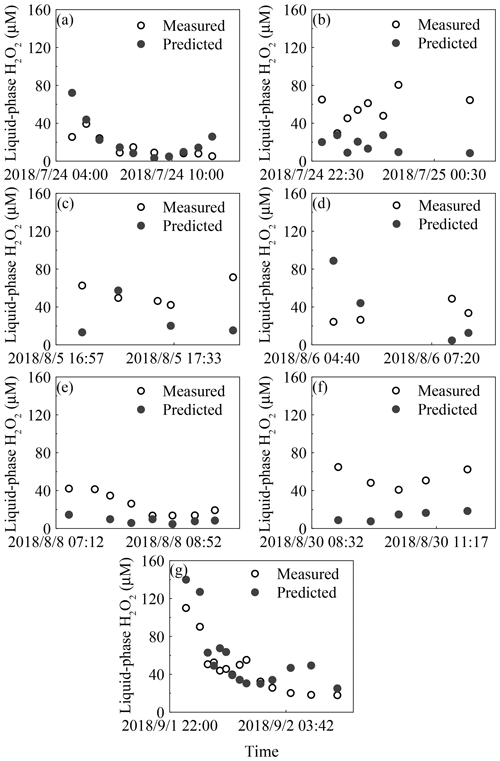Figure 1Time profiles of measured and predicted concentrations of H2O2 from seven rain episodes. The seven rainfall events are listed in chronological order: (a) 24 July, (b) 25 July, (c) 5 August, (d) 6 August, (e) 8 August, (f) 30 August, and (g) 2 September.

To explain the difference between ${H}_{\mathrm{A}}^{\mathrm{m}}$ and ${H}_{\mathrm{A}}^{\mathrm{t}}$, we should rule out the effects of pressure, pH, and TS on ${H}_{\mathrm{A}}^{\mathrm{t}}$. First, to our knowledge, the influence of pressure on ${H}_{\mathrm{A}}^{\mathrm{t}}$ can usually be neglected under conditions of less than 1 atm (Lind and Kok, 1986). Also, ${H}_{\mathrm{A}}^{\mathrm{t}}$ of H2O2 is independent of pH in the range of 4–7 (Xu et al., 2012); therefore, the present study does not consider the influences of pressure and pH on ${H}_{\mathrm{A}}^{\mathrm{t}}$. The temperature during BJ-2018Summer could be divided into three ranges: 294–296, 297–299, and 300–306 K. The percentages of samples in these three temperature ranges were 25 %, 63 %, and 12 %, respectively, and the ratios of ${H}_{\mathrm{A}}^{\mathrm{m}}$ to ${H}_{\mathrm{A}}^{\mathrm{t}}$ in the same temperature ranges were 1.4, 2.6, and 4.5, respectively. The maximum value of ${H}_{\mathrm{A}}^{\mathrm{t}}$ in the range 294–306 K was 1.2×105 M atm−1, while ${H}_{\mathrm{A}}^{\mathrm{m}}$ reached 4.2×105 M atm−1 at the 90th percentile. This suggested that the influence of TS on ${H}_{\mathrm{A}}^{\mathrm{t}}$ was negligible. The non-linear relationship between ${H}_{\mathrm{A}}^{\mathrm{m}}$ and TS, shown in Fig. S3, also indicated that TS played an unimportant role in determining ${H}_{\mathrm{A}}^{\mathrm{m}}$. Thus, other explanations were needed for understanding the difference between ${H}_{\mathrm{A}}^{\mathrm{m}}$ and ${H}_{\mathrm{A}}^{\mathrm{t}}$.

### 3.1.2 Process of raindrops falling

The solubility of H2O2 in clouds is higher than that in the ground rainwater. There is a negative dependence of the solubility on temperature (Huang and Chen, 2010), which allows for the possibility of mass transfer of H2O2 from rainwater to the surrounding air when falling. Let us assume that the gas-phase H2O2 concentration is homogeneous and the rain droplet size remains constant during the falling process. The diameter of the raindrops (DP) is mainly distributed in the range of 0.05–2.50 mm. Calculations were performed for typical droplet diameters at 0.1, 0.5, 1.0, and 2.0 mm. The height of the precipitation cloud base during summer time in north China was always less than 2000 m (Shang et al., 2012), so we assumed the fall distance to be 500, 1000, 1500, and 2000 m, respectively, which remained the same as previous studies (Adamowicz, 1979; Levine and Schwartz, 1982). In the process of falling, it was necessary to consider the mass transfer resistance in the gas and liquid phases. However, it could be that the shear force generated on the raindrop surface when it fell improved the mixing rate in the droplet significantly; therefore, the liquid-phase mass transfer resistance was negligible (Pruppacher and Klett, 1997; Elperin and Fominykh, 2005). Thus, the overall mass transfer resistance reduced to the mass transfer resistance in the gas phase.

Here, we first discussed residual H2O2 in raindrops after a fall from a height of 1000 m. The temperature in clouds (${T}_{\mathrm{S}}^{\mathrm{c}}$) was estimated to be 292 K, which was 6 K lower than the ground. ${H}_{\mathrm{A}}^{\mathrm{t}}$ in pure water at 292 K was 1.4×105 M atm−1 (Sander et al., 2011). Provided that the droplet started at equilibrium with the cloud atmosphere and the level of H2O2 in the cloud atmosphere was equal to the level near the ground (0.30±0.26 ppbv), the initial level of liquid-phase H2O2 before falling (${C}_{\mathrm{aq}}^{\mathrm{0}}$) was 42.87 µM. However, the equilibrium was broken when the raindrops fell as the ambient temperature increased. The mass transfer coefficient in the gas phase (kg) can be calculated by Eqs. (S1) to (S4) in the Supplement (Levine and Schwartz, 1982; Kumar, 1985). The concentration of H2O2 in the droplet at the ground (${C}_{\mathrm{aq}}^{\mathrm{d}}$) can be estimated by Eq. (S5). The results are presented in Table 1, demonstrating that the large droplet has a small mass transfer coefficient. As a result, the liquid-phase H2O2 in the large raindrops was more slowly released into the air. ${C}_{\mathrm{aq}}^{\mathrm{d}}$ of the droplet diameter at 2.0 mm was close to ${C}_{\mathrm{aq}}^{\mathrm{0}}$, while ${C}_{\mathrm{aq}}^{\mathrm{d}}$ at 0.1 mm approximated the theoretical level of liquid-phase H2O2 in pure water at 298 K, as indicated by Fig. 2. The results demonstrated that the effect of residual H2O2 in large raindrops on the level of H2O2 in ground rainwater could be of great importance.

Table 1Calculating the level of H2O2 in the ground raindrops (${C}_{\mathrm{aq}}^{\mathrm{d}}$) with different diameters (DP) from a height of 1000 ma.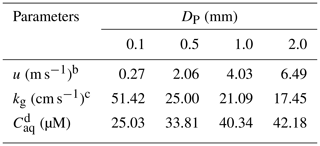a These parameters are calculated based on equations in Gunn and Kinzer (1949), Levine and Schwartz (1982), Kumar (1985), and Seinfeld and Pandis (2006). b u is the terminal fall velocity of a raindrop. c kg is the mass transfer coefficient of H2O2 in the gas phase.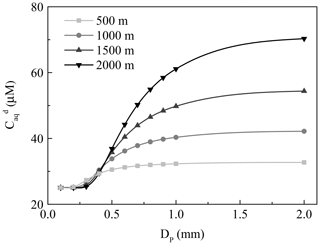Figure 2The dependence of the concentration of H2O2 in the ground raindrops (${C}_{\mathrm{aq}}^{\mathrm{d}}$) on the diameter of the raindrops (DP). The light grey, grey, dark grey, and black lines denote fall distances of 500, 1000, 1500, and 2000 m, respectively.

Next, we investigated the influence of different fall distances on ${C}_{\mathrm{aq}}^{\mathrm{d}}$. The decreasing temperature at increasing fall distances caused larger ${H}_{\mathrm{A}}^{\mathrm{t}}$ and ${C}_{\mathrm{aq}}^{\mathrm{0}}$ in clouds, and ${C}_{\mathrm{aq}}^{\mathrm{d}}$ also increased. The wide gap of ${C}_{\mathrm{aq}}^{\mathrm{d}}$ between different fall distances was more visible for the large droplet, as seen in Fig. 2. Based on the above analysis, the residual H2O2 in large raindrops could increase the H2O2 level in rainwater to a maximum of 48.81 µM at a fall distance of 2000 m, which could explain to a large extent the difference between the measured and predicted levels of H2O2 in rainwater.

Based on the rain intensity, seven rain events during BJ-2018Summer could be divided into three types, as shown in Table S2 in the Supplement. Rain events in types I, II, and III have rain intensities <1, 1–10, and >10 mm h−1, respectively. The larger the diameter of raindrops, the greater the rain intensity (Kumar, 1985). According to the above relationship between the diameter of raindrops and the level of liquid-phase H2O2 in the ground rainwater, the difference between the measured and predicted liquid-phase H2O2 levels should be greater as the hourly rain intensity increases. We found that the differences between ${C}_{\mathrm{aq}}^{\mathrm{m}}$ and ${C}_{\mathrm{aq}}^{\mathrm{t}}$ increased during the rain periods on 25 July and 5 August, during which the maximum hourly rain intensities were more than 10 mm h−1. Because it is difficult for the liquid-phase H2O2 in heavy rains to diffuse into the gas phase, much H2O2 may be retained in the ground rainwater, which could well represent the level of H2O2 in cloud water.

During the rain episode on 1–2 September 2018, the concentration of gas-phase H2O2 decreased over time. However, there was a sudden rise from 0.47 ppbv at 01:03 local time (LT) to 0.66 ppbv at 01:46 LT, which subsequently dropped to 0.38 ppbv over time (Fig. 3a). Surprisingly, the difference between the measured and predicted levels of liquid-phase H2O2 reached a low value in the meantime, indicating that the increase in gas-phase H2O2 was due to the release of H2O2 from raindrops that contained high level of H2O2, as presented in Fig. 3b. Compared with Fig. S4 in the Supplement, which described the relationship between rain intensity and time, the rain intensity simultaneously dropped to 3.51 mm h−1 from 6.35 mm h−1, consequently decreasing the diameter of the raindrops and increasing the mass transfer of H2O2 from rainwater to the gas phase. Provided that 20 µM H2O2 in rainwater was released into ambient air, the increase in the gas-phase H2O2 level was 0.24 ppbv, which was in accordance with the sudden rise during 01:03–01:46 LT on 1–2 September 2018.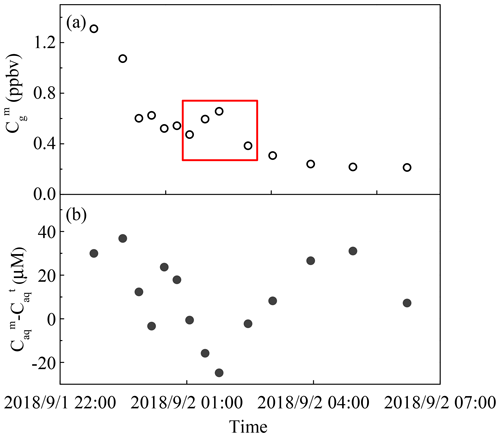Figure 3The measured and predicted H2O2 levels in a rain event on 1–2 September 2018. (a) Measured gas-phase H2O2 (${C}_{\mathrm{g}}^{\mathrm{m}}$). (b) The difference between measured (${C}_{\mathrm{aq}}^{\mathrm{m}}$) and theoretical (${C}_{\mathrm{aq}}^{\mathrm{t}}$) levels of H2O2 in the liquid phase. The red box indicates a sudden rise in gas-phase H2O2.

The above analysis assumes that the gas-phase H2O2 concentration is uniform. However, the distribution of gas-phase H2O2 at different heights may be complicated. We could use the average level of H2O2 in rainwater at the ground to estimate the concentrations of H2O2 in cloud water (${C}_{\mathrm{aq}}^{\mathrm{c}}$) and the nearby atmosphere (${C}_{\mathrm{g}}^{\mathrm{c}}$), as presented in Table 2. Assuming the simplest case, DP is 1.0 mm, the fall distance is 1000 m, and the levels of H2O2 in the gas phase and rainwater at the ground are 0.30 ppbv and 44.12 µM at 298 K, respectively. Considering the release of H2O2 from raindrops into ambient air during the falling process, the level of H2O2 in cloud water should be 47 µM. Based on Henry's law, the surrounding gas-phase H2O2 should be 0.33 ppbv, a little higher than that at the ground. When the fall distance is 500, 1500, and 2000 m, H2O2 in cloud water should be 46, 49, and 51 µM, respectively, and H2O2 in nearby ambient air could be 0.41, 0.26, and 0.21 ppbv, respectively.

Table 2Estimates of the level of H2O2 in cloud water (${C}_{\mathrm{aq}}^{\mathrm{c}}$) and the surrounding atmosphere (${C}_{\mathrm{g}}^{\mathrm{c}}$) at different fall distances with a raindrop diameter of 1.0 mm.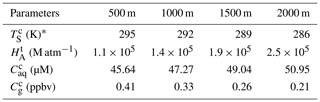${T}_{\mathrm{S}}^{\mathrm{c}}$ is the temperature in cloud water.

## 3.2 Gas–aerosol phase partitioning

### 3.2.1 Gas- and aerosol-phase H2O2 in winter

From 21 December 2018 to 5 January 2019, the gas-phase H2O2 level was 24.08±28.83 pptv, as shown in Fig. S5a in the Supplement. We eluted Teflon filters with H3PO4 solution and measured the level of H2O2 in the extracted solution to calculate the aerosol-phase H2O2 concentration. The mass concentration of aerosol-phase H2O2 and the normalized concentration to aerosol mass were 2.22±1.49 ng m−3 (DL – 6.75 ng m−3) and 0.093±0.085 ng µg−1 (DL – 0.409 ng µg−1), respectively. The mean concentration of the aerosol-phase H2O2 at night-time (0.107±0.102 ng µg−1) was higher than that at daytime (0.079±0.066 ng µg−1), as presented in Fig. S5b. The level of aerosol-phase H2O2 in the present study was lower than those reported in previous studies (Table S3), which may be due to the extraction method, the extraction time, reducing substance levels, or aerosol pH value, as shown in the Supplement. Assuming a molecular weight of 300 g mol−1 (Docherty et al., 2005; Epstein et al., 2014), the level of TPOs averaged 10.26±6.38 ng µg−1 (2.08–28.75 ng µg−1). It was calculated that H2O2 took up a small fraction of TPOs, equal to 8±6 % in molar concentration ratio, which indicated that organic peroxides accounted for a large proportion of peroxides and could play important roles in the formation of PM2.5 and secondary organic aerosols.

The measured level of H2O2 in aerosols was much higher than the predicted value using Pankow's absorptive partitioning theory, which suggested that the aerosols collected on the filter existed under non-equilibrium conditions, and the aerosol-phase H2O2 may arise from sources other than gas-phase partitioning. Based on Eqs. (3) and (4), ${K}_{\mathrm{P}}^{\mathrm{m}}$ was equal to $\mathrm{3.8}×{\mathrm{10}}^{-\mathrm{3}}±\mathrm{4.8}×{\mathrm{10}}^{-\mathrm{3}}$ m3µg−1 at 270±4 K, which was 4 orders of magnitude higher than ${K}_{\mathrm{P}}^{\mathrm{t}}$, $\mathrm{2.8}×{\mathrm{10}}^{-\mathrm{7}}$ m3µg−1. The effect of parameter variation on calculating ${K}_{\mathrm{P}}^{\mathrm{t}}$ could not account for the large discrepancy between ${K}_{\mathrm{P}}^{\mathrm{m}}$ and ${K}_{\mathrm{P}}^{\mathrm{t}}$ (Shen et al., 2018), and other factors are needed to explain the difference. In terms of the proportion of theoretical to measured concentrations, the partitioning of gas-phase H2O2 into aerosols could be neglected, and nearly all of the aerosol-phase H2O2 was generated from other reactions.

Because aerosol water content (AWC) cannot be correctly evaluated at low RH, the effective field-derived Henry's law constant (${H}_{\mathrm{P}}^{\mathrm{m}}$) of H2O2 was estimated for the high-RH condition, e.g. a heavy haze episode from 2 to 3 January 2019 (RH, 30 %). Details regarding the estimation of AWC are shown in the Supplement. It was calculated that AWC, ${C}_{\mathrm{p}}^{\mathrm{m}}$, and ${C}_{\mathrm{g}}^{\mathrm{m}}$ levels averaged 3.20 µg m−3, 6.63×103µM, and $\mathrm{1.90}×{\mathrm{10}}^{-\mathrm{11}}$ atm. Based on Eq. (5), the average ${H}_{\mathrm{P}}^{\mathrm{m}}$ on 2–3 January 2019 was calculated to be $\mathrm{2.7}×{\mathrm{10}}^{\mathrm{8}}±\mathrm{1.8}×{\mathrm{10}}^{\mathrm{8}}$ M atm−1. However, the theoretical Henry's law constant (${H}_{\mathrm{P}}^{\mathrm{t}}$) at 270 K was 1.1×106 M atm−1 (Sander et al., 2011), which was lower than ${H}_{\mathrm{P}}^{\mathrm{m}}$ by 2 orders of magnitude. In the study of Chung et al. (2005), the salting-in effect can improve the level of H2O2 by a factor of 2 when the concentrations in salt solutions were up to 10 M, and the most obvious salting-in effect of salt solutions was ammonium sulfate. In this paper, the levels of aerosol-phase ${\mathrm{NH}}_{\mathrm{4}}^{+}$ and ${\mathrm{SO}}_{\mathrm{4}}^{\mathrm{2}-}$ on 2–3 January 2019 were 94 and 21 M, respectively, and the level of (NH4)2SO4 was assumed to be 21 M. The increase of ${H}_{\mathrm{P}}^{\mathrm{m}}$ by the salting-in effect of (NH4)2SO4 was about 3.2×106 M atm−1 at 286 K based on equations in Chung et al. (2005). Even though aerosol particles were collected at 270±4 K and the increase may be greater, the salting-in effect could not fully explain the difference between ${H}_{\mathrm{P}}^{\mathrm{m}}$ and ${H}_{\mathrm{P}}^{\mathrm{t}}$. Other sources need to be found later.

### 3.2.2 Factor analysis

Figure 4 demonstrates that the concentration of aerosol-phase H2O2 is dependent on RH, with a trend that is first increasing (stage A) and then decreasing (stage B) as RH increases. The variation of H2O2 with RH was the result of competition between production and consumption processes. Here, the production process refers to either process that favours increasing the level of aerosol-phase H2O2, while the consumption process refers to the process consuming aerosol-phase H2O2. In the first stage, the higher RH could accelerate the heterogeneous uptake of H2O2 onto aerosols and enhance the level of aerosol-phase H2O2 (Pradhan et al., 2010; Shiraiwa et al., 2011; Zhao et al., 2013; Slade and Knopf, 2015). The level of H2O2 was negatively associated with RH in the subsequent stage, ascribed to much more rapid consumption of H2O2 due to its oxidizing of the reducing substances on polluted days, such as SO2 into ${\mathrm{SO}}_{\mathrm{4}}^{\mathrm{2}-}$.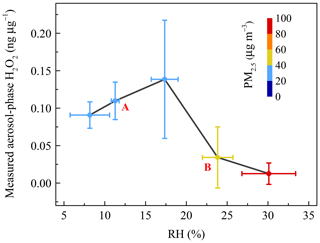Figure 4The relationship between measured aerosol-phase H2O2 level and relative humidity (RH). Coloured circles denote the mass concentration of PM2.5. The vertical error bars represent the standard deviations of aerosol-phase H2O2 concentration in every RH range bin. A and B refer to the increase and decrease stages, respectively.

We considered a heavy haze episode, from 2 to 3 January 2019, as an example to explain the important contribution of aerosol-phase H2O2 to ${\mathrm{SO}}_{\mathrm{4}}^{\mathrm{2}-}$ growth on polluted days in detail. The PM2.5 mass concentration of the severe haze event was up to 201.20 µg m−3. Based on the measured H2O2, the reaction rate (RR) and sulfate formation rate (SFR) averaged about $\mathrm{3.03}×{\mathrm{10}}^{-\mathrm{3}}$µmol m−3 h−1 and 0.29 µg m−3 h−1 (Table S4), respectively. The detailed calculation process is provided in the Supplement. In addition, the growth rate of ${\mathrm{SO}}_{\mathrm{4}}^{\mathrm{2}-}$ calculated by the measured data was 0.51 µg m−3 h−1, and H2O2 oxidation pathway contributed about 57 % of the measured growth of ${\mathrm{SO}}_{\mathrm{4}}^{\mathrm{2}-}$ in PM2.5. This result strongly suggested that the aerosol-phase H2O2 indeed acted as an important oxidant in the formation of sulfate, and played significant roles in the rapid growth of PM2.5 during pollution events.

Next, we considered that the consumption rate of aerosol-phase H2O2 increased with an increase of RH. The extent of the concentration variations of H2O2, ${\mathrm{SO}}_{\mathrm{4}}^{\mathrm{2}-}$, and PM2.5 at 10th and 90th percentiles were 22, 6, and 5, respectively, suggesting that the inverse relationship still existed when we eliminated the interference of the dilution effect due to a high aerosol loading. The dilution effect was unimportant and could be neglected. It is suggested that larger levels of ${\mathrm{SO}}_{\mathrm{4}}^{\mathrm{2}-}$ and PM2.5 are often accompanied by higher RH. In Fig. 5, the reverse curve between aerosol-phase ${\mathrm{SO}}_{\mathrm{4}}^{\mathrm{2}-}$ and H2O2 became steeper with the formation of ${\mathrm{SO}}_{\mathrm{4}}^{\mathrm{2}-}$, indicating that the rate of consumption of H2O2 on polluted days was much higher than that on clean days, which could offer proof of rapid H2O2 consumption with increasing RH. In addition, the level of H2O2 in the aerosol phase exhibited a negative correlation with PM2.5 mass concentration, as shown in Fig. 5. In other words, the aerosol-phase H2O2 concentration was lower on polluted days than on clean days, further demonstrating that the removal rate of H2O2 by oxidizing SO2 into ${\mathrm{SO}}_{\mathrm{4}}^{\mathrm{2}-}$ exceeded the production rate during pollution events with high RH.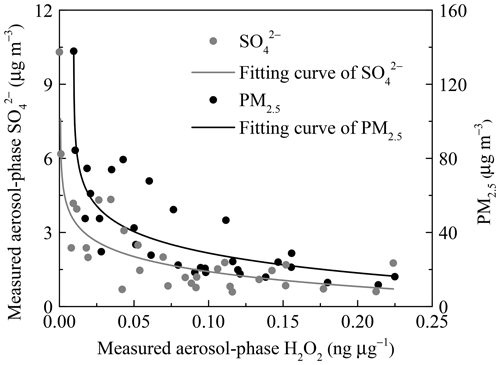Figure 5The negative dependence of the measured concentrations of aerosol-phase ${\mathrm{SO}}_{\mathrm{4}}^{\mathrm{2}-}$ and PM2.5 on H2O2. The grey and black lines are the logarithmic fits for ${\mathrm{SO}}_{\mathrm{4}}^{\mathrm{2}-}$ level in aerosols and the PM2.5 mass concentration, respectively.

### 3.2.3 Heterogeneous uptake of H2O2 and HO2

In addition to the factors that influence the aerosol-phase H2O2 concentration, there are certain physical and chemical reactions other than gas-phase partitioning that could increase the level of aerosol-phase H2O2, e.g. heterogeneous uptake of H2O2 on aerosols. Previous studies have shown that heterogeneous uptake of H2O2 is positively related to RH. High RH is beneficial to the mass transfer of H2O2 from the gas phase to the aerosol phase, which could accelerate the reaction between H2O2 and reducing compositions of aerosols, thus contributing to more heterogeneous uptake of H2O2 (Huang et al., 2015; Wu et al., 2015). To quantitatively evaluate the importance of the heterogeneous uptake of H2O2 on aerosols to the aerosol-phase H2O2, we calculated the average rate of heterogeneous uptake based on Eqs. (S6) to (S11) in the Supplement. Details about each parameter were introduced in Table 3, and the heterogeneous uptake rate of H2O2 ($\mathrm{d}\left[X{\right]}_{\mathrm{p}}^{\mathrm{t},\mathrm{h}}/\mathrm{d}t\right)$ averaged 0.02 ng µg−1 h−1.

Table 3Calculating the theoretical heterogeneous uptake rate of H2O2 on aerosols ($\mathrm{d}\left[X{\right]}_{\mathrm{p}}^{\mathrm{t},\mathrm{h}}/\mathrm{d}t$)a.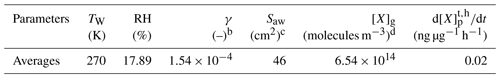a These parameters are calculated based on Wu et al. (2015). b γ is the heterogeneous uptake coefficient (dimensionless). c Saw is the surface area of aerosols, quoted from Kuang et al. (2019). d [X]g is the concentration of gas-phase H2O2.

As HO2 radical is a precursor of H2O2, the heterogeneous uptake of HO2 onto aerosols may also contribute to the formation of the aerosol-phase H2O2. We assumed that the reactive uptake coefficient of HO2 to aerosol particles was 0.2, and the product of HO2 was H2O2 (Li et al., 2019). At the same observation site in winter of 2017, HO2 concentration for noontime averaged $\left(\mathrm{0.4}±\mathrm{0.2}\right)×{\mathrm{10}}^{\mathrm{8}}$ and $\left(\mathrm{0.3}±\mathrm{0.2}\right)×{\mathrm{10}}^{\mathrm{8}}$ cm−3 on clean and polluted days, respectively (Ma et al., 2019). Since HO2 level data in 2018 was not available, we used the level of HO2 on clean days in winter of 2017 for calculations, and the average was about 0.2×108 cm−3 at daytime. The heterogeneous uptake rate of HO2 on aerosols was calculated in the same way as H2O2, and the formation rate of the aerosol-phase H2O2 by reactive uptake of HO2 averaged 0.22 ng µg−1 h−1 all day.

### 3.2.4 Decomposition of organic peroxides

It was demonstrated that the concentration of H2O2 in the extracted solution first increased rapidly, then reached peaks at distinct hours that depended on the specific sample, and finally declined gradually over time. However, interestingly, there was large sample-to-sample variation, with samples classifiable into three types in terms of the change in trend and evolution duration (Fig. 6 and Table 4). The third type (Fig. 6c) occurred when the H2O2 level exhibited a steady decline without a growth stage within 13 h, and this was the case with samples 5 and 6 on a slightly polluted day on 2 January 2019. Samples 1 and 2 on 29 December 2018 during clean days belonged to the first type (Fig. 6a), during which H2O2 rapidly grew within 5 h and subsequently decreased at a slow rate over 25 h. The evolution trends of H2O2 in the second type (samples 3 and 4, Fig. 6b) during clean days from 31 December 2018 to 1 January 2019 were similar to those of the first type, except H2O2 approached its peak at about 40 h over the whole analysis process lasting for about 300 h. Given that the H2O2 concentration increased in the extracted solution as time went, the effects of the extraction and transportation processes on the effective gas-aerosol partitioning coefficient of H2O2 were discussed, as shown in the Supplement.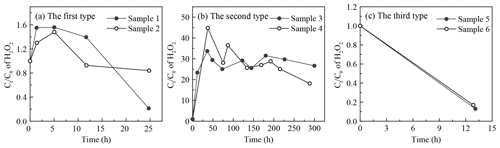Figure 6Time profiles of aerosol-phase H2O2 evolution in the extracted solution. (a) The first type: samples 1 and 2 were collected on 29 December 2018. (b) The second type: samples 3 and 4 were gathered on 31 December 2018–1 January 2019. (c) The third type: samples 5 and 6 were collected on 2 January 2019. Ct and C0 denote molar concentrations of H2O2 in the extracted solution at time =t and time =0.

Table 4Comparison of the H2O2 evolution parameters in the extracted solution among the three types.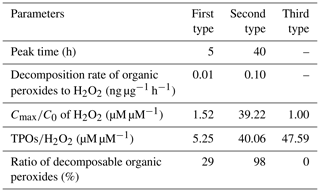To seek the reasons for the elevated level of H2O2 in the extracted solution, we compared the ratio of the maximum (Cmax) to initial (C0) H2O2 concentrations in the extracted solution with the molar concentration ratio of the aerosol-phase TPOs to H2O2 and found that the ratios of ${C}_{max}/{C}_{\mathrm{0}}$ and TPOs∕H2O2 were in the same order of magnitude for the first and second types, as exhibited in Table S5 in the Supplement. This result provided evidence that part of the aerosol-phase H2O2 originated from the decomposition/hydrolysis of organic peroxides, as described in earlier studies (Wang et al., 2011; Li et al., 2016). In the second type, the concentration of TPOs normalized to aerosol mass reached a maximum, indicating that the second type had more TPO sources, which consequently caused higher TPOs∕H2O2 and ${C}_{max}/{C}_{\mathrm{0}}$ ratios compared with the first type. Furthermore, the aerosol surface is semi-liquid or liquid under high RH (Liu et al., 2017), which provides reaction sites for the decomposition/hydrolysis of aerosol-phase organic peroxides. Aerosol-phase organic peroxides could decompose into H2O2 before the particle aerosols were collected (Zhao et al., 2018). Thus, the decomposition/hydrolysis of organic peroxides in the extracted solution could be applied to the ambient particles. The average rates of the decomposition/hydrolysis of organic peroxides to H2O2 in the rising stage for the first and second types were 0.01 and 0.10 ng µg−1 h−1, respectively.

The three types of samples were in accordance with the growth process of PM2.5. According to meteorological parameters and trace-gas data (Table S6 in the Supplement), static weather conditions gradually formed and were accompanied by lower wind speed, lower ozone level, higher RH, and higher gaseous pollutants levels. The mass concentration of PM2.5 increased from 13.45 to 63.11 µg m−3. In addition, the mass concentration of TPOs also showed a rising trend, whereas the level of TPOs normalized to aerosol mass increased at first and decreased afterwards due to the rapid growth of PM2.5. Because of the consumption of reactive TPOs, which formed ${\mathrm{SO}}_{\mathrm{4}}^{\mathrm{2}-}$ during polluted days, the rest of the TPOs were stable organic peroxides that could not easily decompose into H2O2, e.g. peroxide esters (ROOR). The ratio of TPOs∕H2O2 in the third type, collected on a slightly polluted day, was close to that in the second type on clean days, but a rising trend of H2O2 in the extracted solution could not be observed. It was calculated that the ratios of decomposable TPOs to total TPOs for the three types were 29 %, 98 %, and 0 %, respectively.

Recently, it was reported that organic peroxides accounted for a large proportion of secondary organic aerosol (SOA) mass, varying widely from less than 20 % to 60 % (Docherty et al., 2005; Li et al., 2016; Gong et al., 2018). Peroxy radicals also played important parts in the formation of highly oxygenated molecules (HOMs) via an autoxidation mechanism, which can form aerosols without sulfuric acid nucleation (Kirkby et al., 2016). The thermal decomposition of peroxide-containing SOAs and HOMs contributed to the formation of aerosol-phase H2O2 (Krapf et al., 2016). A similar phenomenon was also found by Li et al. (2016), in which the decomposition/hydrolysis of organic peroxides sustainably generated H2O2 accompanied by the attenuation of TPOs in the extracted solution, and about 18 % of gaseous organic peroxides underwent the heterogeneous decomposition on aerosols into H2O2. The decomposable organic peroxides are often peroxycarboxylic acids (PCAs, e.g. peroxyacetic acid, PAA; peroxyformic acid, PFA) and α-hydroxyalkyl-hydroperoxides (α-HAHPs, e.g. hydroxymethyl hydroperoxide, HMHP).

## 3.3 Source and sink of H2O2 in rainwater and aerosols

To provide support for the sources suggested above, we analysed the sources and sinks of liquid- and aerosol-phase H2O2 in rainwater and aerosols. In this study, the measured level of H2O2 was the concentration after partial or complete reaction with reducing substances, such as SO2 onto the particles. The contribution of different additional sources in the liquid and aerosol phases should be estimated compared with the important sink.

The level of liquid-phase H2O2 was the result of the combined effect between sources (gas-phase partitioning and residual H2O2 in raindrops) and sinks (reaction with S(IV) and the decomposition of H2O2). Based on the foregoing description, the dissolved gas-phase H2O2 in rainwater was 25.20 µM at 298 K. The residual H2O2 in raindrops could enhance the level of liquid-phase H2O2 by up to 48.81 µM. The largest removal pathway of liquid-phase H2O2 was to oxidize dissolved SO2 into sulfate. Given that the major oxidants to sulfate formation were only H2O2 and O3 (Penkett et al., 1979; Chandler et al., 1988), the proportions of the H2O2 oxidation pathway to the overall, calculated by Eqs. (S12) and (S13) in the Supplement, were 92 % at pH 5 and 11 % at pH 6, respectively. The average of sulfate concentration in rainwater was measured to be 31.95 µM, and the H2O2 oxidation pathway contributed to the sulfate with 29 µM at pH 5 and 4 µM at pH 6, which was the consumption molar concentration of H2O2. In addition, the decomposition of H2O2 during 6 h storage time before analysis was 6 µM (Li et al., 2016). To summarize, the concentration of liquid-phase H2O2 was supposed to have its maximum at 64.01 µM, a bit lower than the 90th percentile of the measured level (67.85 µM). This could be considered to achieve the approximate balance between sources and sinks in the liquid-phase H2O2. Consequently, the residual H2O2 in raindrops could explain the difference between ${H}_{\mathrm{A}}^{\mathrm{m}}$ and ${H}_{\mathrm{A}}^{\mathrm{t}}$.

With respect to the sources and sinks for aerosol-phase H2O2, the main removal pathway was the consumption of H2O2 to sulfate formation, similar to the sink of H2O2 in the liquid phase. The average mass concentrations of PM2.5 and aerosol-phase ${\mathrm{SO}}_{\mathrm{4}}^{\mathrm{2}-}$ were 39.21 and 2.20 µg m−3, respectively. The mass concentration ratio of ${\mathrm{SO}}_{\mathrm{4}}^{\mathrm{2}-}$ to PM2.5 was 6 % in this study, which was lower than previous studies (Ho et al., 2016; Shao et al., 2018). The discrepancy may be explained by the decreased ratio of ${\mathrm{SO}}_{\mathrm{4}}^{\mathrm{2}-}$ to PM2.5 due to SO2 emission control in recent years, as shown in the Supplement.

We estimated the contribution of different sources to the aerosol-phase H2O2 based on the formation and consumption rates. According to the previous estimation of the theoretical sulfate formation rate (0.29 µg m−3 h−1) and the average mass concentration of PM2.5 (106.19 µg m−3) from 2 to 3 January 2019, the consumption rate of H2O2 should be 0.97 ng µg−1 h−1. With respect to the sources of the aerosol-phase H2O2, the decomposition/hydrolysis of organic peroxides was firstly considered, with average rates of the rising stage for the first and second types (Fig. 6), 0.01 and 0.10 ng µg−1 h−1, respectively. Because the extracted solution was stored under 255 K, lower than the actual atmospheric temperature (270 K), the decomposition/hydrolysis rates of organic peroxides were underestimated and an adjusting factor should be multiplied. The factors for the three typical labile organic peroxides (HMHP, PFA, and PAA) were 13, 3, and 2, respectively, as shown in the Supplement. Assuming the factor was in the range of 2–13, the average decomposition/hydrolysis rate of organic peroxides for the first and second types (0.055 ng µg−1 h−1) was used to calculate the formation rate. The formation rate of the aerosol-phase H2O2 from the decomposition/hydrolysis of organic peroxides could account for 11 %–74 % of the consumption rate by sulfate formation. Moreover, the heterogeneous uptake of HO2 and H2O2 was also likely to improve the aerosol-phase H2O2 level at the rates of 0.22 and 0.02 ng µg−1 h−1, respectively, which can offset 22 % and 2 % of the consumption rate of H2O2, respectively. The source and sink rates of H2O2 did not seem to reach a balance. In our view, there were a few possible explanations for the difference. First, we estimated the contribution of the H2O2 oxidation pathway to sulfate formation during the entire measurement period based on the contribution of high-pollution days, which may overestimate the sink for the aerosol-phase H2O2. Second, the inverse dependence of the γ value on the gas-phase H2O2 concentration was not considered, and the γ value could be underestimated when the level of gas-phase H2O2 in winter was much lower (Romanias et al., 2012, 2013). Third, there may be missing sources in aerosol-phase H2O2 which are not completely understood.

Based on the above analysis, sources and sinks of H2O2 in the liquid phase could achieve a balance, while the formation of H2O2 from the decomposition/hydrolysis of aerosol-phase organic peroxides and the heterogeneous uptake of HO2 and H2O2 could not offset the consumption of H2O2 in the aerosol phase. Field measurements and laboratory experiments are urgently needed to further study the possible reasons and search for missing sources of aerosol-phase H2O2.

4 Conclusions

In this study, we simultaneously measured H2O2 concentrations in gas and rainwater in summer as well as in the gas and aerosol phases (PM2.5) in winter over urban Beijing. For the seven investigated rain episodes, the average ${H}_{\mathrm{A}}^{\mathrm{m}}$ was 2.1×105 M atm−1, which was 2.5 times greater than ${H}_{\mathrm{A}}^{\mathrm{t}}$ at 298±2 K. The liquid-phase concentration of H2O2 averaged 44.12±26.49µM. In 69 % of the rain samples, the liquid-phase H2O2 level was much higher than the predicted value estimated for pure water using Henry's law. We found that 12 % of measured H2O2 in all samples and 59 % of measured H2O2 in those samples with high level of measured liquid-phase H2O2 were from residual H2O2 in raindrops. With an increase in fall distance, the proportion of the additional source of liquid-phase H2O2 gradually increased. In addition, the sink of H2O2 due to droplet-to-gas transfer was reduced with an increase in raindrop diameter; thus, the liquid-phase H2O2 level also increased. Furthermore, the source and sink of H2O2 in rainwater could achieve a balance.

For the measured PM2.5 aerosol samples, a similar phenomenon was observed between the measured and predicted levels of H2O2 in the aerosol phase, but the difference was much higher than that in the liquid phase. ${K}_{\mathrm{P}}^{\mathrm{m}}$ averaged $\mathrm{3.8}×{\mathrm{10}}^{-\mathrm{3}}$ m3µg−1, which was 4 orders of magnitude higher than ${K}_{\mathrm{P}}^{\mathrm{t}}$ at 270±4 K. The aerosol-phase concentration of H2O2 normalized to the aerosol mass averaged 0.093±0.085 ng µg−1. The decomposition/hydrolysis of organic peroxides produced the elevated aerosol-phase H2O2 at a maximum rate of 0.10 ng µg−1 h−1, responsible for 11 %–74 % of the consumption rate of aerosol-phase H2O2, and its value depended on the composition of organic peroxides in the aerosol particles. The heterogeneous uptake of HO2 and H2O2 played a minor role in increasing the H2O2 level in the aerosol phase, and the proportions based on the consumption rate of H2O2 were 22 % and 2 %, respectively. There are many uncertainties in the decomposition/hydrolysis of organic peroxides in this study, and laboratory simulation studies are needed to quantify the roles of different organic peroxides in the decomposition process. Aerosol-phase H2O2 in this study cannot reach source and sink equilibrium, and there are missing sources of aerosol-phase H2O2. Due to a lack of substantial severe haze events with high RH in this study, the source and sink mentioned in the aerosol-phase H2O2 need to be further verified.

Our study has provided direct evidence to prove that the partitioning of H2O2 between the gas-liquid and gas-aerosol phases not only follows thermodynamic equilibrium but is also affected by certain physical and chemical reactions. The effective field-derived Henry's law constant and gas-aerosol partitioning coefficient should be accepted to better predict the measured liquid- and aerosol-phase H2O2 concentrations, which would be beneficial to correctly calculating the contribution of H2O2 to the fast formation of ${\mathrm{SO}}_{\mathrm{4}}^{\mathrm{2}-}$ and PM2.5 during pollution episodes. More laboratory experiments and field measurements are urgently needed to improve our understanding of the partitioning of peroxides in different phases in the atmosphere.

Data availability

The data are accessible by contacting the corresponding author (zmchen@pku.edu.cn).

Supplement

Author contributions

In the framework of BJ-2018Summer and BJ-2018Winter measurements, ZC and XX designed the study. XX carried out all peroxide measurements used in this study, analysed the data, and wrote the paper. ZC helped interpret the results, guided the writing, and modified the manuscript. YG contributed to the methods of analysing aerosol-phase hydrogen peroxide and total peroxides. HS helped interpret data and modified the manuscript. SC provided the data for the meteorological parameters, trace gases, and PM2.5 mass concentrations. All authors discussed the results and contributed to the final paper.

Competing interests

The authors declare that they have no conflict of interest.

Acknowledgements

We gratefully thank the editor and two anonymous reviewers for their constructive suggestions that helped us to improve the article.

Financial support

This research has been supported by the National Key Research and Development Program of China (grant no. 2016YFC0202704), the National Research Program for Key Issues in Air Pollution Control (grant no. DQGG0103), and the National Natural Science Foundation of China (grant no. 21477002).

Review statement

This paper was edited by Nga Lee Ng and reviewed by two anonymous referees.

References

Adamowicz, R. F.: A model for the reversible washout of sulfur-dioxide, ammonia and carbon-dioxide from a polluted atmosphere and the production of sulfates in raindrops, Atmos. Environ., 13, 105–121, https://doi.org/10.1016/0004-6981(79)90250-6, 1979.

Arellanes, C., Paulson, S. E., Fine, P. M., and Sioutas, C.: Exceeding of Henry's law by hydrogen peroxide associated with urban aerosols, Environ. Sci. Technol., 40, 4859–4866, https://doi.org/10.1021/es0513786, 2006.

Banerjee, D. K. and Budke, C. C.: Spectrophotometric determination of traces of peroxides in organic solvents, Anal. Chem., 36, 792–796, https://doi.org/10.1021/ac60210a027, 1964.

Baum, E. J.: Chemical property estimation: theory and application, CRC Press LLC, Florida, USA, 1998.

Campbell, S. J., Stevanovic, S., Miljevic, B., Bottle, S. E., Ristovski, Z., and Kalberer, M.: Quantification of particle-bound organic radicals in secondary organic aerosol, Environ. Sci. Technol., 53, 6729–6737, https://doi.org/10.1021/acs.est.9b00825, 2019.

Chandler, A. S., Choularton, T. W., Dollard, G. J., Eggleton, A. E. J., Gay, M. J., Hill, T. A., Jones, B. M. R., Tyler, B. J., Bandy, B. J., and Penkett, S. A.: Measurements of H2O2 and SO2 in clouds and estimates of their reaction rate, Nature, 336, 562–565, https://doi.org/10.1038/336562a0, 1988.

Charrier, J. G., McFall, A. S., Richards-Henderson, N. K., and Anastasio, C.: Hydrogen peroxide formation in a surrogate lung fluid by transition metals and quinones present in particulate matter, Environ. Sci. Technol., 48, 7010–7017, https://doi.org/10.1021/es501011w, 2014.

Chung, M. Y., Muthana, S., Paluyo, R. N., and Hasson, A. S.: Measurements of effective Henry's law constants for hydrogen peroxide in concentrated salt solutions, Atmos. Environ., 39, 2981–2989, https://doi.org/10.1016/j.atmosenv.2005.01.025, 2005.

Crowley, J. N., Pouvesle, N., Phillips, G. J., Axinte, R., Fischer, H., Petäjä, T., Nölscher, A., Williams, J., Hens, K., Harder, H., Martinez-Harder, M., Novelli, A., Kubistin, D., Bohn, B., and Lelieveld, J.: Insights into HOx and ROx chemistry in the boreal forest via measurement of peroxyacetic acid, peroxyacetic nitric anhydride (PAN) and hydrogen peroxide, Atmos. Chem. Phys., 18, 13457–13479, https://doi.org/10.5194/acp-18-13457-2018, 2018.

Docherty, K. S., Wu, W., Lim, Y. B., and Ziemann, P. J.: Contributions of organic peroxides to secondary aerosol formed from reactions of monoterpenes with O3, Environ. Sci. Technol., 39, 4049–4059, https://doi.org/10.1021/es050228s, 2005.

Elperin, T. and Fominykh, A.: Conjugate mass transfer during gas absorption by falling liquid droplet with internal circulation, Atmos. Environ., 39, 4575–4582, https://doi.org/10.1016/j.atmosenv.2005.04.005, 2005.

Epstein, S. A., Blair, S. L., and Nizkorodov, S. A.: Direct photolysis of α-pinene ozonolysis secondary organic aerosol: effect on particle mass and peroxide content, Environ. Sci. Technol., 48, 11251–11258, https://doi.org/10.1021/es502350u, 2014.

Gong, Y., Chen, Z., and Li, H.: The oxidation regime and SOA composition in limonene ozonolysis: roles of different double bonds, radicals, and water, Atmos. Chem. Phys., 18, 15105–15123, https://doi.org/10.5194/acp-18-15105-2018, 2018.

Gunn, R. and Kinzer, G. D.: The terminal velocity of fall for water droplets in stagnant air, J. Meteorol., 6, 243–248, https://doi.org/10.1175/1520-0469(1949)006<0243:TTVOFF>2.0.CO;2, 1949.

Gurgueira, S. A., Lawrence, J., Coull, B., Murthy, G. G. K., and González-Flecha, B.: Rapid increases in the steady-state concentration of reactive oxygen species in the lungs and heart after particulate air pollution inhalation, Environ. Health Perspect., 110, 749–755, https://doi.org/10.1289/ehp.02110749, 2002.

Hasson, A. S. and Paulson, S. E.: An investigation of the relationship between gas-phase and aerosol-borne hydroperoxides in urban air, J. Aerosol Sci., 34, 459–468, https://doi.org/10.1016/S0021-8502(03)00002-8, 2003.

He, S. Z., Chen, Z. M., Zhang, X., Zhao, Y., Huang, D. M., Zhao, J. N., Zhu, T., Hu, M., and Zeng, L. M.: Measurement of atmospheric hydrogen peroxide and organic peroxides in Beijing before and during the 2008 Olympic Games: chemical and physical factors influencing their concentrations, J. Geophys. Res., 115, D17307, https://doi.org/10.1029/2009JD013544, 2010.

Ho, K. F., Ho, S. S. H., Huang, R. J., Chuang, H. C., Cao, J. J., Han, Y. M., Lui, K. H., Ning, Z., Chuang, K. J., Cheng, T. J., Lee, S. C., Hu, D., Wang, B., and Zhang, R. J.: Chemical composition and bioreactivity of PM2.5 during 2013 haze events in China, Atmos. Environ., 126, 162–170, https://doi.org/10.1016/j.atmosenv.2015.11.055, 2016.

Hua, W., Chen, Z. M., Jie, C. Y., Kondo, Y., Hofzumahaus, A., Takegawa, N., Chang, C. C., Lu, K. D., Miyazaki, Y., Kita, K., Wang, H. L., Zhang, Y. H., and Hu, M.: Atmospheric hydrogen peroxide and organic hydroperoxides during PRIDE-PRD'06, China: their concentration, formation mechanism and contribution to secondary aerosols, Atmos. Chem. Phys., 8, 6755–6773, https://doi.org/10.5194/acp-8-6755-2008, 2008.

Huang, D. M. and Chen, Z. M.: Reinvestigation of the Henry's law constant for hydrogen peroxide with temperature and acidity variation, J. Environ. Sci., 22, 570–574, https://doi.org/10.1016/S1001-0742(09)60147-9, 2010.

Huang, L. B., Zhao, Y., Li, H., and Chen, Z. M.: Kinetics of heterogeneous reaction of sulfur dioxide on authentic mineral dust: effects of relative humidity and hydrogen peroxide, Environ. Sci. Technol., 49, 10797–10805, https://doi.org/10.1021/acs.est.5b03930, 2015.

Kirkby, J., Duplissy, J., Sengupta, K., Frege, C., Gordon, H., Williamson, C., Heinritzi, M., Simon, M., Yan, C., Almeida, J., Tröstl, J., Nieminen, T., Ortega, I. K., Wagner, R., Adamov, A., Amorim, A., Bernhammer, A. K., Bianchi, F., Breitenlechner, M., Brilke, S., Chen, X. M., Craven, J., Dias, A., Ehrhart, S., Flagan, R. C., Franchin, A., Fuchs, C., Guida, R., Hakala, J., Hoyle, C. R., Jokinen, T., Junninen, H., Kangasluoma, J., Kim, J., Krapf, M., Kürten, A., Laaksonen, A., Lehtipalo, K., Makhmutov, V., Mathot, S., Molteni, U., Onnela, A., Peräkylä, O., Piel, F., Petäjä, T., Praplan, A. P., Pringle, K., Rap, A., Richards, N. A. D., Riipinen, I., Rissanen, M. P., Rondo, L., Sarnela, N., Schobesberger, S., Scott, C. E., Seinfeld, J. H., Sipilä, M., Steiner, G., Stozhkov, Y., Stratmann, F., Tomé, A., Virtanen, A., Vogel, A. L., Wagner, A. C., Wagner, P. E., Weingartner, E., Wimmer, D., Winkler, P. M., Ye, P. L., Zhang, X., Hansel, A., Dommen, J., Donahue, N. M., Worsnop, D. R., Baltensperger, U., Kulmala, M., Carslaw, K. S., and Curtius, J.: Ion-induced nucleation of pure biogenic particles, Nature, 533, 521–526, https://doi.org/10.1038/nature17953, 2016.

Krapf, M., EI Haddad, I., Bruns, E. A., Molteni, U., Daellenbach, K. R., Prévôt, A. S. H., Baltensperger, U., and Dommen, J.: Labile peroxides in secondary organic aerosol, Chem, 1, 603–616, https://doi.org/10.1016/j.chempr.2016.09.007, 2016.

Kuang, Y., Tao, J. C., Xu, W. Y., Yu, Y. L., Zhao, G., Shen, C. Y., Bian, Y. X., and Zhao, C. S.: Calculating ambient aerosol surface area concentrations using aerosol light scattering enhancement measurements, Atmos. Environ., 216, 116919, https://doi.org/10.1016/j.atmosenv.2019.116919, 2019.

Kumar, S.: An Eulerian model for scavenging of pollutants by raindrops, Atmos. Environ., 19, 769–778, https://doi.org/10.1016/0004-6981(85)90065-4, 1985.

Lee, M. H., Heikes, B. G., and O'Sullivan, D. W.: Hydrogen peroxide and organic hydroperoxide in the troposphere: a review, Atmos. Environ., 34, 3475–3494, https://doi.org/10.1016/S1352-2310(99)00432-X, 2000.

Levine, S. Z. and Schwartz, S. E.: In-cloud and below-cloud scavenging of nitric acid vapor, Atmos. Environ., 16, 1725–1734, https://doi.org/10.1016/0004-6981(82)90266-9, 1982.

Li, H., Chen, Z., Huang, L., and Huang, D.: Organic peroxides' gas-particle partitioning and rapid heterogeneous decomposition on secondary organic aerosol, Atmos. Chem. Phys., 16, 1837–1848, https://doi.org/10.5194/acp-16-1837-2016, 2016.

Li, K., Jacob, D. J., Liao, H., Zhu, J., Shah, V., Shen, L., Bates, K. H., Zhang, Q., and Zhai, S. X.: A two-pollutant strategy for improving ozone and particulate air quality in China, Nat. Geosci., 12, 906–910, https://doi.org/10.1038/s41561-019-0464-x, 2019.

Li, T., Wang, Z., Wang, Y., Wu, C., Liang, Y., Xia, M., Yu, C., Yun, H., Wang, W., Wang, Y., Guo, J., Herrmann, H., and Wang, T.: Chemical characteristics of cloud water and the impacts on aerosol properties at a subtropical mountain site in Hong Kong SAR, Atmos. Chem. Phys., 20, 391–407, https://doi.org/10.5194/acp-20-391-2020, 2020.

Liang, H., Chen, Z. M., Huang, D., Zhao, Y., and Li, Z. Y.: Impacts of aerosols on the chemistry of atmospheric trace gases: a case study of peroxides and HO2 radicals, Atmos. Chem. Phys., 13, 11259–11276, https://doi.org/10.5194/acp-13-11259-2013, 2013.

Lind, J. A. and Kok, G. L.: Henry's law determinations for aqueous solutions of hydrogen peroxide, methylhydroperoxide, and peroxyacetic acid, J. Geophys. Res., 91, 7889–7895, https://doi.org/10.1029/JD091iD07p07889, 1986.

Liu, T. Y., Clegg, S. L., and Abbatt, J. P. D.: Fast oxidation of sulfur dioxide by hydrogen peroxide in deliquesced aerosol particles, P. Natl. Acad. Sci. USA, 117, 1354–1359, https://doi.org/10.1073/pnas.1916401117, 2020.

Liu, Y. C., Wu, Z. J., Wang, Y., Xiao, Y., Gu, F. T., Zheng, J., Tan, T. Y., Shang, D. J., Wu, Y. S., Zeng, L. M., Hu, M., Bateman, A. P., and Martin, S. T.: Submicrometer particles are in the liquid state during heavy haze episodes in the urban atmosphere of Beijing, China, Environ. Sci. Technol. Lett., 4, 427–432, https://doi.org/10.1021/acs.estlett.7b00352, 2017.

Ma, X. F., Tan, Z. F., Lu, K. D., Yang, X. P., Liu, Y. H., Li, S. L., Li, X., Chen, S. Y., Novelli, A., Cho, C. M., Zeng, L. M., Wahner, A., and Zhang, Y. H.: Winter photochemistry in Beijing: observation and model simulation of OH and HO2 radicals at an urban site, Sci. Total Environ., 685, 85–95, https://doi.org/10.1016/j.scitotenv.2019.05.329, 2019.

Maass, O. and Hiebert, P. G.: The properties of pure hydrogen peroxide. v. vapor pressure, J. Am. Chem. Soc., 46, 2693–2700, https://doi.org/10.1021/ja01677a012, 1924.

Nozaki, K.: Iodometric method of analysis for organic peroxides, Ind. Eng. Chem., 18, 583–583, https://doi.org/10.1021/i560157a020, 1946.

O'Sullivan, D. W., Lee, M., Noone, B. C., and Heikes, B. G.: Henry's law constant determinations for hydrogen peroxide, methyl hydroperoxide, hydroxymethyl hydroperoxide, ethyl hydroperoxide, and peroxyacetic acid, J. Phys. Chem., 100, 3241–3247, https://doi.org/10.1021/jp951168n, 1996.

Pankow, J. F.: An absorption model of gas-particle partitioning of organic compounds in the atmosphere, Atmos. Environ., 28, 185–188, https://doi.org/10.1016/1352-2310(94)90093-0, 1994.

Penkett, S. A., Jones, B. M. R., Brich, K. A., and Eggleton, A. E. J.: The importance of atmospheric ozone and hydrogen peroxide in oxidising sulphur dioxide in cloud and rainwater, Atmos. Environ., 13, 123–137, https://doi.org/10.1016/j.atmosenv.2007.10.065, 1979.

Pradhan, M., Kyriakou, G., Archibald, A. T., Papageorgiou, A. C., Kalberer, M., and Lambert, R. M.: Heterogeneous uptake of gaseous hydrogen peroxide by Gobi and Saharan dust aerosols: a potential missing sink for H2O2 in the troposphere, Atmos. Chem. Phys., 10, 7127–7136, https://doi.org/10.5194/acp-10-7127-2010, 2010.

Pruppacher, H. R. and Klett, J. D.: Microphysics of clouds and precipitation, Kluwer Academic Publishers, Dordrecht, the Netherlands, 1997.

Qin, M. R., Chen, Z. M., Shen, H. Q., Li, H., Wu, H. H., and Wang, Y.: Impacts of heterogeneous reactions to atmospheric peroxides: observations and budget analysis study, Atmos. Environ., 183, 144–153, https://doi.org/10.1016/j.atmosenv.2018.04.005, 2018.

Qiu, J., Ishizuka, S., Tonokura, K., Colussi, A. J., and Enami, S.: Water dramatically accelerates the decomposition of α-hydroxyalkyl-hydroperoxides in aerosol particles, J. Phys. Chem. Lett., 10, 5748–5755, https://doi.org/10.1021/acs.jpclett.9b01953, 2019.

Reeves, C. E. and Penkett, S. A.: Measurements of peroxides and what they tell us, Chem. Rev., 103, 5199–5218, https://doi.org/10.1021/cr0205053, 2003.

Riva, M., Budisulistiorini, S. H., Zhang, Z. F., Gold, A., Thornton, J. A., Turpin, B. J., and Surratt, J. D.: Multiphase reactivity of gaseous hydroperoxide oligomers produced from isoprene ozonolysis in the presence of acidified aerosols, Atmos. Environ., 152, 314–322, https://doi.org/10.1016/j.atmosenv.2016.12.040, 2017.

Romanias, M. N., El Zein, A., and Bedjanian, Y.: Heterogeneous interaction of H2O2 with TiO2 surface under dark and UV light irradiation conditions, J. Phys. Chem. A, 116, 8191–8200, https://doi.org/10.1021/jp305366v, 2012.

Romanias, M. N., El Zein, A., and Bedjanian, Y.: Uptake of hydrogen peroxide on the surface of Al2O3 and Fe2O3, Atmos. Environ., 77, 1–8, https://doi.org/10.1016/j.atmosenv.2013.04.065, 2013.

Sander, S. P., Abbatt, J., Barker, J. R., Burkholder, J. B., Friedl, R. R., Golden, D. M., Huie, R. E., Kolb, C. E., Kurylo, M. J., Moortgat, G. K., Orkin, V. L., and Wine, P. H.: Chemical kinetics and photochemical data for use in atmospheric studies, Evaluation No. 17, JPL Publication 10-6, Jet Propulsion Laboratory, Pasadena, USA, available at: http://jpldataeval.jpl.nasa.gov (last access: 19 March 2020), 2011.

Seinfeld, J. H. and Pandis S. N.: Atmospheric chemistry and physics: from air pollution to climate change, 2nd Edition, John Wiley and Sons, New Jersey, USA, 2006.

Shang, B., Zhou, Y. Q., Liu, J. C., and Huang, Y. M.: Comparing vertical structure of precipitation cloud and non-precipitation cloud using Cloudsat, J. Appl. Meteor. Sci., 23, 1–9, https://doi.org/10.3969/j.issn.1001-7313.2012.01.001, 2012.

Shao, P. Y., Tian, H. Z., Sun, Y. J., Liu, H. J., Wu, B. B., Liu, S. H., Liu, X. Y., Wu, Y. M., Liang, W. Z., Wang, Y., Gao, J. J., Xue, Y. F., Bai, X. X., Liu, W., Lin, S. M., and Hu, G. Z.: Characterizing remarkable changes of severe haze events and chemical compositions in multi-size airborne particles (PM1, PM2.5 and PM10) from January 2013 to 2016–2017 winter in Beijing, China, Atmos. Environ., 189, 133–144, https://doi.org/10.1016/j.atmosenv.2018.06.038, 2018.

Shen, H., Barakat, A. I., and Anastasio, C.: Generation of hydrogen peroxide from San Joaquin Valley particles in a cell-free solution, Atmos. Chem. Phys., 11, 753–765, https://doi.org/10.5194/acp-11-753-2011, 2011.

Shen, H. Q., Chen, Z. M., Li, H., Qian, X., Qin, X., and Shi, W. X.: Gas-particle partitioning of carbonyl compounds in the ambient atmosphere, Environ. Sci. Technol., 52, 10997–11006, https://doi.org/10.1021/acs.est.8b01882, 2018.

Shiraiwa, M., Ammann, M., Koop, T., and Pöschl, U.: Gas uptake and chemical aging of semisolid organic aerosol particles, P. Natl. Acad. Sci. USA, 108, 11003–11008, https://doi.org/10.1073/pnas.1103045108, 2011.

Slade, J. H. and Knopf, D. A.: Multiphase OH oxidation kinetics of organic aerosol: the role of particle phase state and relative humidity, Geophys. Res. Lett., 41, 5297–5306, https://doi.org/10.1002/2014GL060582, 2015.

Stein, A. F. and Saylor, R. D.: Sensitivities of sulfate aerosol formation and oxidation pathways on the chemical mechanism employed in simulations, Atmos. Chem. Phys., 12, 8567–8574, https://doi.org/10.5194/acp-12-8567-2012, 2012.

Tong, H., Arangio, A. M., Lakey, P. S. J., Berkemeier, T., Liu, F., Kampf, C. J., Brune, W. H., Pöschl, U., and Shiraiwa, M.: Hydroxyl radicals from secondary organic aerosol decomposition in water, Atmos. Chem. Phys., 16, 1761–1771, https://doi.org/10.5194/acp-16-1761-2016, 2016.

Wang, Y., Kim, H., and Paulson, S. E.: Hydrogen peroxide generation from α- and β-pinene and toluene secondary organic aerosols, Atmos. Environ., 45, 3149–3156, https://doi.org/10.1016/j.atmosenv.2011.02.060, 2011.

Wang, Y., Chen, Z., Wu, Q., Liang, H., Huang, L., Li, H., Lu, K., Wu, Y., Dong, H., Zeng, L., and Zhang, Y.: Observation of atmospheric peroxides during Wangdu Campaign 2014 at a rural site in the North China Plain, Atmos. Chem. Phys., 16, 10985–11000, https://doi.org/10.5194/acp-16-10985-2016, 2016.

Williams, B. J., Goldstein, A. H., Kreisberg, N. M., and Hering, S. V.: In situ measurements of gas/particle-phase transitions for atmospheric semivolatile organic compounds, P. Natl. Acad. Sci. USA, 107, 6676–6681, https://doi.org/10.1073/pnas.0911858107, 2010.

Wu, Q. Q., Huang, L. B., Liang, H., Zhao, Y., Huang, D., and Chen, Z. M.: Heterogeneous reaction of peroxyacetic acid and hydrogen peroxide on ambient aerosol particles under dry and humid conditions: kinetics, mechanism and implications, Atmos. Chem. Phys., 15, 6851–6866, https://doi.org/10.5194/acp-15-6851-2015, 2015.

Xie, M. J., Hannigan, M. P., and Barsanti, K. C.: Gas/particle partitioning of n-alkanes, PAHs and oxygenated PAHs in urban Denver, Atmos. Environ., 95, 355–362, https://doi.org/10.1016/j.atmosenv.2014.06.056, 2014.

Xu, Z. F., Tang, Y., and Ji, J. P.: Chemical and strontium isotope characterization of rainwater in Beijing during the 2008 Olympic year, Atmos. Res., 107, 115–125, https://doi.org/10.1016/j.atmosres.2012.01.002, 2012.

Ye, C., Liu, P. F., Ma, Z. B., Xue, C. Y., Zhang, C. L., Zhang, Y. Y., Liu, J. F., Liu, C. T., Sun, X., and Mu, Y. J.: High H2O2 concentrations observed during haze periods during the winter in Beijing: importance of H2O2 oxidation in sulfate formation, Environ. Sci. Technol. Lett., 5, 757–763, https://doi.org/10.1021/acs.estlett.8b00579, 2018.

Zhao, R., Kenseth, C. M., Huang, Y., Dalleska, N. F., and Seinfeld, J. H.: Iodometry-assisted liquid chromatography electrospray ionization mass spectrometry for analysis of organic peroxides: an application to atmospheric secondary organic aerosol, Environ. Sci. Technol., 52, 2108–2117, https://doi.org/10.1021/acs.est.7b04863, 2018.

Zhao, Y., Chen, Z. M., Shen, X. L., and Zhang, X.: Kinetics and mechanisms of heterogeneous reaction of gaseous hydrogen peroxide on mineral oxide particles, Environ. Sci. Technol., 45, 3317–3324, https://doi.org/10.1021/es104107c, 2011.

Zhao, Y., Chen, Z. M., Shen, X. L., and Huang, D.: Heterogeneous reactions of gaseous hydrogen peroxide on pristine and acidic gas-processed calcium carbonate particles: effects of relative humidity and surface coverage of coating, Atmos. Environ., 67, 63–72, https://doi.org/10.1016/j.atmosenv.2012.10.055, 2013.

Zhou, X. L., Davis, A. J., Kieber, D. J., Keene, W. C., Maben, J. R., Maring, H., Dahl, E. E., Izaguirre, M. A., Sander, R., and Smoydzyn, L.: Photochemical production of hydroxyl radical and hydroperoxides in water extracts of nascent marine aerosols produced by bursting bubbles from Sargasso seawater, Geophys. Res. Lett., 35, L20803, https://doi.org/10.1029/2008GL035418, 2008.